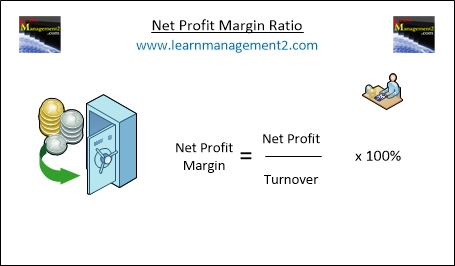# Net Profit Margin

The Net Profit Margin compares the net profit figure with sales (turnover). The net profit margin shows what percentage of the sales (or turnover) is net profit. The net profit margin calculation is very similar to gross profit margin, the only difference is that you use net profit instead of gross profit in the calculation.This diagram shows how to calculate net profit margin using the net profit and turnover figures

### How To Calculate The Net Profit Margin

Use the following method to work out the net profit margin:

Net Profit                     Net Profit
Margin             =          _________      x 100%

Turnover

### Example Net Profit Margin Calculation

Let’s use the previous example – My turnover is £10000 and my gross profit is £6000. When I subtracted expenses that were not related to sales and added non sales income (to my gross profit), I was left with £4000 net profit. To work out net profit margin I would use the following calculation:

Net Profit                     £4000
_________      =          _____              x 100   = 40% Net Profit Margin

Turnover                     £10000

### Comparing Gross Profit Margin With Net Profit Margin

If I compare gross profit margin with net profit margin it should enable me to investigate how non sale expenses and income are affecting a firm's profit margin. In my example gross profit margin is 60% and net profit margin is 40%. This means that income and expenses that are not directly related to sales has reduced my profit margin by 20 percent. As a business it is worth looking at net and gross profit margin to make sure that overheads (non cost of sale expenses) aren't reducing profit margins more than you would like.

### Conclusion

We hope you enjoyed learning how to calculate net profit margin. Here are some related articles to help you continue learning about profitability ratios

Related Articles : Profitability Ratios Overview | Gross Profit Margin | Return On Capital Employed